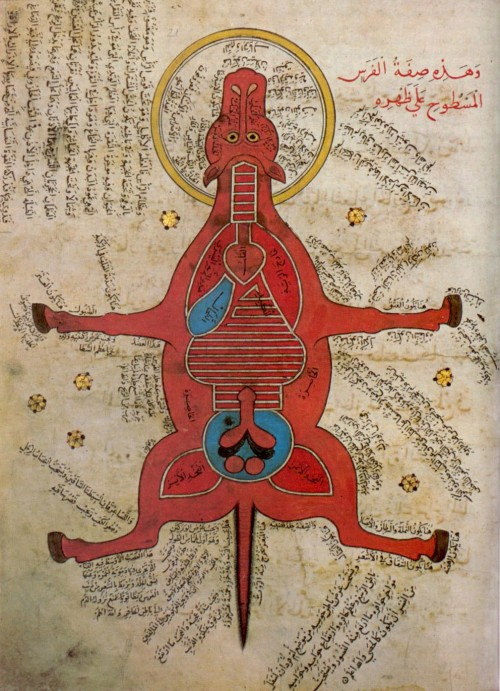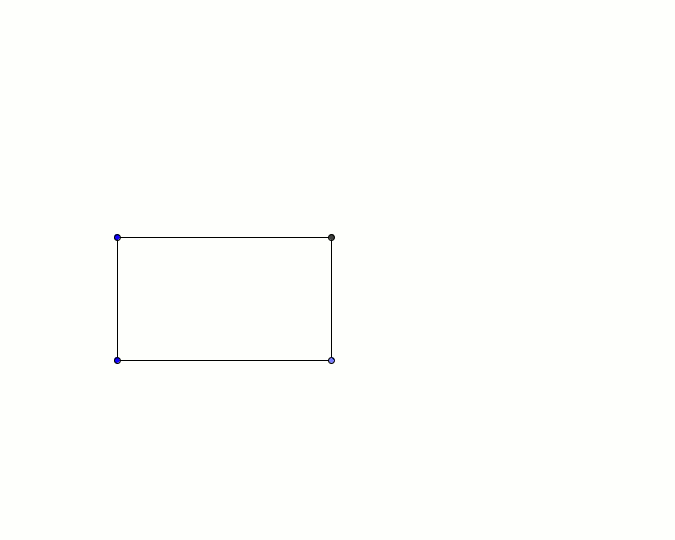Tag: diagramAnatomy of a horse from a 15th century AD Egyptian document at the University Library, Istanbul.This is the construction of a square that shares the area of a given rectangle. The red means two things: if there is only one red line on the frame, it means “This line is the radius that the just constructed circle has.” If there are two or more red lines on the frame, then it means “These radii must be equal to each other.”By Dr. Monster
Travis Pitts